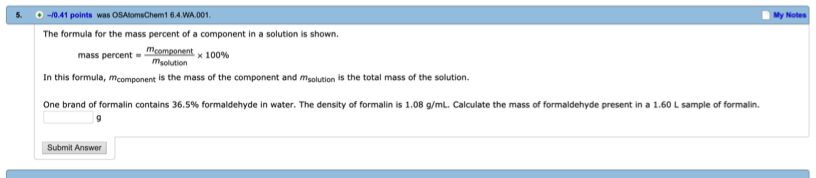# The formula for the mass percent of a component in a solution is shown. mass percentmcomponent = x 100% msolution In this formula, mcomponent is the mass of the component and msolution is the total mass of the solution. One brand of formalin contains 36.5% formaldehyde in water. The density of formalin is 1.08 g/mL. Calculate the mass of formaldehyde present in a 1.60 L sample of formalin